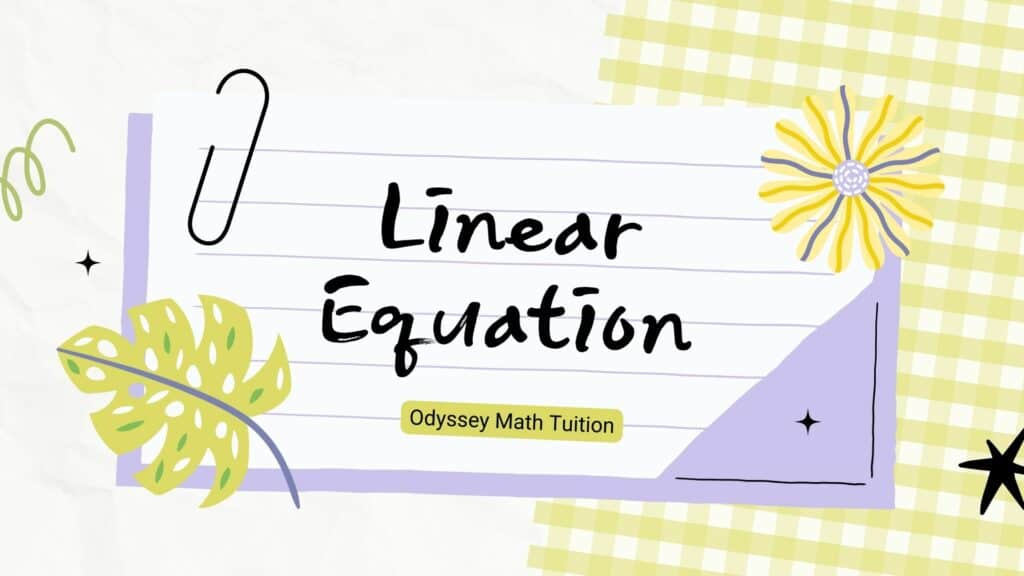# Linear Equations

### Linear Equations

Linear Equation is an equation that forms a straight line when it is graphed. They are written in the form of y=mx+c. Always remember that in the equation y=mx+c, m is the gradient (rise divided by run) and c is the vertical-intercept.

Now that you understand a little more about linear equations, journey with us and check out non linear equations next!